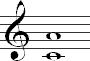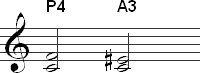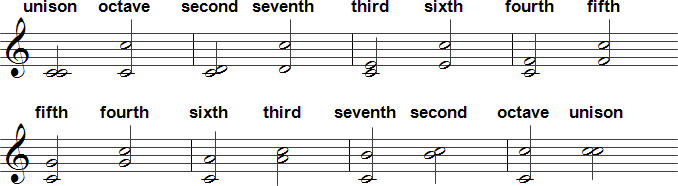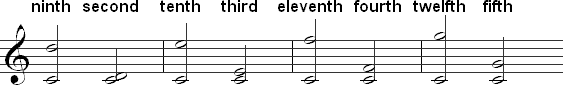What You Will Learn
1. How to classify intervals
2. What enharmonic intervals are
3. What compound intervals are
4. How to invert intervals

How to Identify Intervals

What is an Interval?

An interval is the distance in pitch between two notes. Intervals are named according to the number of notes (letter names) and half-steps between the two notes.

Melodic and Harmonic Intervals

Intervals can be either melodic or harmonic. Melodic intervals occur when the notes involved are played separately, as in a melody. Harmonic intervals are when the notes are played at the same time.

Classifying Intervals

Interval Names

Interval names consist of two parts:

• a number for the size of the interval (unison, second, third, fourth, fifth, sixth, seventh, or octave)
• a prefix for the quality of the interval (major, minor, perfect, diminished, or augmented)

Here are a few examples of possible interval names using the conventions above:

• minor third
• perfect fourth
• augmented sixth

The interval is almost always determined by starting on the lower pitch. All examples in this lesson work this way, but there are situations where you may need to determine an interval below a pitch. For example, you may need to transpose music down by a certain interval to play it in a different key.

Interval Number

The number or size of an interval can be determined by counting the number of letter names between the two pitches. You should begin counting with the number one on the lower pitch. Try this with the example below:The interval from C to A includes six pitches (C, D, E, F, G, A), so it is a sixth. Keep in mind that there are seven letters in the music alphabet from A to G, so if you reach G, you start over with A until you reach the second note in the interval.

The following chart shows the basic interval names and how many letter names are in each:

Interval name Number of letters
unison 1
second 2
third 3
fourth 4
fifth 5
sixth 6
seventh 7
octave 8

Note that the unison and octave are usually referred to using these names rather than a numerical value, such as first or eighth.

Here is an example of each of these intervals starting on C:Interval Quality

The prefix part of an interval name can be determined by counting the number of half-steps between the two pitches. Each of these prefixes will only be used with certain intervals. Major and minor will only be used with seconds, thirds, sixths, and sevenths, while perfect will only be used with unisons, fourths, fifths, and octaves. Augmented and diminished can be used with any interval. The chart below summarizes details about interval quality.

Interval quality Description Used with
Augmented One half step larger than either a major or perfect interval any interval
Diminished One half step smaller than either a minor or perfect interval any interval
Major One half step larger than a minor interval seconds, thirds, sixths, and sevenths
Minor One half step smaller than a major interval seconds, thirds, sixths, and sevenths
Perfect n/a unisons, fourths, fifths, octaves

Double Augmented and Diminished Intervals

It is possible to have a doubly augmented or diminished interval, but these occur rarely. A doubly augmented interval will be one half step larger than an augmented interval, while a doubly diminished interval will be one half step smaller than a diminished interval.

Shorthand Notation for Interval Quality

These prefixes are often written in shorthand followed by a number for the numerical value of the interval:

• A for augmented
• d for diminished
• M for major
• m for minor
• P for perfect

Note the capitalization of each prefix. Minor and diminished should be indicated with lower case letters. The other prefixes should be capitalized. The chart below lists common intervals and the shorthand notation for each of them.

Shorthand Interval
m2 minor second
M2 major second
m3 minor third
M3 major third
P4 perfect fourth
A4 augmented fourth
d5 diminished fifth
P5 perfect fifth
m6 minor sixth
M6 major sixth
m7 minor seventh
M7 major seventh

Note that unisons and octaves can also be written in shorthand by using a "1" for a unison and an "8" for an octave. However, unisons and octaves are generally called by these names rather than the shorthand.

Determining the Quality of an Interval

The quality of an interval is determined by the number of half steps between the two notes. The chart below gives the number of half steps in the most common intervals.

Interval # of Half Steps
unison 0
minor second 1
major second 2
minor third 3
major third 4
perfect fourth 5
augmented fourth 6
diminished fifth 6
perfect fifth 7
minor sixth 8
major sixth 9
minor seventh 10
major seventh 11
octave 12

Enharmonic Intervals

Enharmonic intervals are intervals that sound the same although they are notated differently. They will have the same number of half-steps, but the number of notes will be different. An example of enharmonic intervals is shown below:Both of the intervals sound the same and have the same number of half steps (5), but each interval has a different number of notes in terms of the letter names between the bottom and top note. The first interval is a perfect fourth. There are four letter names in this interval (C D E F) and five half steps. The second interval is an augmented third. The interval contains three letter names (C D E) and five steps. Although these intervals sound the same, it is important to distinguish between them by using the correct name. The name of the interval is very important in constructing and analyzing things like chords and scales, so make sure to name each interval correctly.

The Tritone

The tritone is the most common enharmonic interval. Tritone is another name for an augmented fourth. The name 'tritone' comes from the three (tri-) whole tones (tone) in the augmented fourth. Although the diminished fifth technically doesn't contain three whole tones, the word tritone is used to describe it as well, since a diminished fifth and augmented fourth are enharmonic intervals.

The tritone can be written in shorthand as A4 or d5 depending on the notes involved, but "TT" is another shorthand for the tritone that is used frequently.

Interval Inversions

Intervals can be inverted by raising the lower pitch by an octave. The quality and size of the interval always follows a certain pattern when inverted. For example, if a third is inverted it is always a sixth.

Inversions: Size

Table of Inversions by Size

The table below lists the inversion of each interval when inverting according to size.

Intervals Inversion
Unison Octave
Second Seventh
Third Sixth
Fourth Fifth
Fifth Fourth
Sixth Third
Seventh Second
Octave Unison

Examples of Inverting by Size

The following example shows how each of these intervals are inverted using C as the bottom note. The first interval is the original interval. The second interval in each measure is the inversion.Inversions: Quality

Table of Inversions by Quality

The following table gives the inversion of each quality: augmented, diminished, major, minor, and perfect. Like inversions of size, these inversion are always the same.

Quality Inversion
Augmented Diminished
Diminished Augmented
Major Minor
Minor Major
Perfect Perfect

Sample Inversions by Quality above a C

An example of each quality inversion is shown below.Simple and Compound Intervals

Simple intervals are an octave or smaller. Compound intervals are intervals that are larger than an octave. Intervals of a ninth, tenth, eleventh, twelfth, and thirteenth are the most common compound intervals. These intervals can have the same qualities as their simple interval counterparts.

Comparing Simple and Compound Intervals

Every compound interval corresponds to a simple interval. A compound interval can be thought of as a simple interval where one of the notes has been raised or lowered by one or more octaves. Subtracting seven from a compound interval will give you the interval number of the simple interval. For example, to determine the simple interval equivalent of a tenth you subtract seven from ten to find that the equivalent is a third. Below are several compound intervals with the corresponding simple interval shown next to each one:The chart below shows the interval name, the number of half steps in each intervals, and the equivalent simple interval for the most commonly used compound intervals:

Compound intervals Half steps Simple interval equivalent
minor ninth 13 minor second
major ninth 14 major second
minor tenth 15 minor third
major tenth 16 major third
perfect eleventh 17 perfect fourth
perfect twelfth 19 fifth
minor thirteenth 20 minor sixth
major thirteenth 21 major sixth

Compound intervals are important in chord construction, so it is essential that you understand the concept. Intervals of a ninth, eleventh, and thirteenth are commonly used in extended chords.